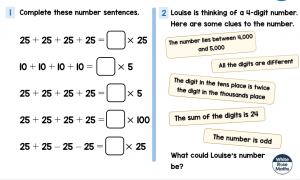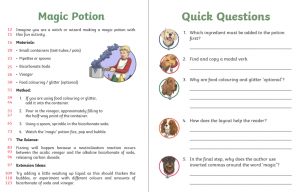# Friday 27th March- Year 5

Ola!  Buenas dias!

Who took part in the applause at 8 o’clock last night?  Wasn’t it lovely?

Maths-English-Spanish-

https://www.languagesonline.org.uk/Hotpotatoes/spanishindex.html

Have a go at one of the tasks-  maybe saying hello, colours or numbers?

Let me know how you go!

### 12 thoughts on “Friday 27th March- Year 5”

•27th March 2020 at 10:04 am

maths,
1.
25+25+25+25=3×25
10+10+10+10=8×5
25+25+25+25=20×5
25+25+25+25=1×100
25+25-25-25=1×25

2.
4389

•27th March 2020 at 11:19 am

Double check the first and last questions Jessica.

•27th March 2020 at 10:33 am

English,

what was the first ingreedent;bicarbonate soda

why did he use food colouring/glitter;to make the potion have colour
what effect did the layout do to the reader;so they know what part they are on and so they don’t loose where they are
why did they put inverted commas around the word ‘magic’ ;because it makes the word’magic’ more powerful and lots of people don’t believe in ‘magic’

•27th March 2020 at 3:27 pm

Jess- double check what was the first thing to put into the container?

Did you spot the modal verb- try the last paragraph!

So if it’s not “magic” what is it?

•27th March 2020 at 11:02 am

25 +25+25+25 =100 ×25 =1,25
10+10+10+10 =40×5=160
25+25+25+25=100 ×5 500
25+25+25+25=100 ×100 = 1,000
25+25+25+25 = 100 × 25=2,525

•27th March 2020 at 11:20 am

Double check this George. Remember what the equals sign means – both sides of it need to be balanced and equal. Are yours?

•27th March 2020 at 2:54 pm

Hello Mrs Leeming and Mrs Millard,
We have emailed Tanisa’s work across on the year 5 email.
ThNk you

•27th March 2020 at 3:28 pm

Hi, I’ve emailed back! Her work is looking very organised!!

•27th March 2020 at 11:55 am

English-

2. Will is the modal verb.
3. It is because you don’t have to put them in.
4. It’s chronological.
5.Because it’s not real magic it is just for excitment.

•27th March 2020 at 3:29 pm

If it’s not magic, what is it?

•27th March 2020 at 5:26 pm

maths
1.25+25=50 25+25=50 50+50=100 100 x 25=2500
2.10+10+10+10=40 40×5 =80
3.25 +25+25+25=100 x 100=10000
4.25 +25 -25-25=0 0x25=0

•